Courses

# Reasoning MCQ - 20

## 30 Questions MCQ Test Reasoning Aptitude for Competitive Examinations | Reasoning MCQ - 20

Description
This mock test of Reasoning MCQ - 20 for LR helps you for every LR entrance exam. This contains 30 Multiple Choice Questions for LR Reasoning MCQ - 20 (mcq) to study with solutions a complete question bank. The solved questions answers in this Reasoning MCQ - 20 quiz give you a good mix of easy questions and tough questions. LR students definitely take this Reasoning MCQ - 20 exercise for a better result in the exam. You can find other Reasoning MCQ - 20 extra questions, long questions & short questions for LR on EduRev as well by searching above.
QUESTION: 1

Solution:
QUESTION: 2

Solution:
QUESTION: 3

### Directions : Read the given information carefully and answer the given questions. Each of the six friends P, Q, R, S, T and U has a different weight. R is heavier than T but lighter than U. Q is lighter than only P. T is not the lightest. T weighs 50 kg. The one who is the third heaviest weighs 63 kg. Q.  Whose weight could possibly be 49 kg?

Solution:
QUESTION: 4

Directions : Read the given information carefully and answer the given questions.

Each of the six friends P, Q, R, S, T and U has a different weight. R is heavier than T but lighter than U. Q is lighter than only P. T is not the lightest. T weighs 50 kg. The one who is the third heaviest weighs 63 kg.

Q.

Which of the following weights correctly represents possible weight of R?

Solution:
QUESTION: 5

How many such pairs of letters are there in the word SCHEDULE, each of which has as many letters between them in the word (in both forward and backward directions) as they have between them in the English alphabetical series?

Q.

Solution: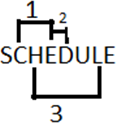QUESTION: 6

Directions : Study the following information and answer the given question:

Point F is 10 m to the south of E. Point G is 3 m to the east of F. Point H is 5 m to the south of G. Point I is 6 m to the west of H. Point J is 10 m to the north of I. Point K is 6 m to the east of J. Point L is 5 m to the north of K.

Q.

Which of the following represents the direction of point G with respect to point J?

Solution: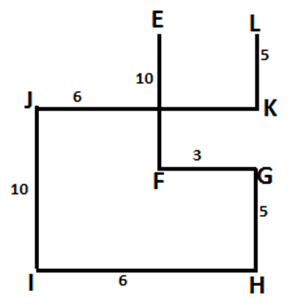QUESTION: 7

Directions : Study the following information and answer the given question:

Point F is 10 m to the south of E. Point G is 3 m to the east of F. Point H is 5 m to the south of G. Point I is 6 m to the west of H. Point J is 10 m to the north of I. Point K is 6 m to the east of J. Point L is 5 m to the north of K.

Q.

How far and in which direction in point E from point L?

Solution:QUESTION: 8

Point R is 10 metres north of point A. Point K is exactly in the middle of the points R and A. Point N is 7 metres east of point A. Point M is 7 metres east of point K. Point S is 6 metres north of point M. What is the distance between points S and N?

Q.

Solution: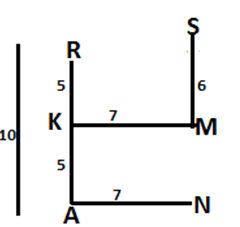QUESTION: 9

A student starts from the school, walks 2 km towards North, takes a left turn and walks for 5 km. He then takes a left turn and walks for 8 km before taking a left turn again and walks for further 5 km then finally takes a left turn and walks 1 km before reaching his home. How far and towards which direction should the student walk to reach the school again?

Q.

Solution: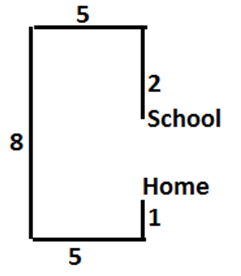QUESTION: 10

Town D is 13 km towards the East of town A. A bus starts from town A, travels 8 km towards West and takes a right turn. After taking the right turn, it travels 5 km and reaches town B. From town B the bus takes a right turn again, travels 21 km and stops. How far and towards which direction must the bus travel to reach town D?

Q.

Solution: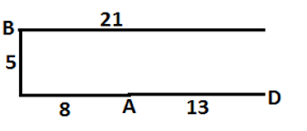QUESTION: 11

Directions : Study the following information and answer the questions given below:

(i) ‘P ÷ Q’ means ‘P is sister of Q’.

(ii) ‘P × Q’ means ‘P is brother of Q’.

(iii) ‘P – Q’ means ‘P is mother of Q’.

(iv) ‘P + Q’ means ‘P is father of Q’.

Q.

Which of the following means ‘M is maternal uncle of T?

Solution: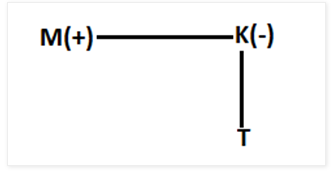QUESTION: 12

Directions : Study the following information and answer the questions given below:

(i) ‘P ÷ Q’ means ‘P is sister of Q’.

(ii) ‘P × Q’ means ‘P is brother of Q’.

(iii) ‘P – Q’ means ‘P is mother of Q’.

(iv) ‘P + Q’ means ‘P is father of Q’.

Q.

Which of the following means ‘H is paternal grandfather of T?

Solution: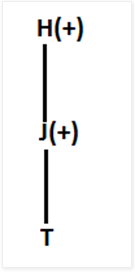QUESTION: 13

Directions : Study the following information carefully and answer the questions given below:

(i) ‘P × Q’ means ‘P is sister of Q’.

(ii) ‘P + Q’ means ‘P is mother of Q’.

(iii) ‘P – Q’ means ‘P is father of Q’.

(iv) ‘P ÷ Q’ means ‘P is brother of Q’

Q.

Which of the following represents ‘W is grandfather of H’?

Solution:
QUESTION: 14

Directions : Study the following information carefully and answer the questions given below:

(i) ‘P × Q’ means ‘P is sister of Q’.

(ii) ‘P + Q’ means ‘P is mother of Q’.

(iii) ‘P – Q’ means ‘P is father of Q’.

(iv) ‘P ÷ Q’ means ‘P is brother of Q’

Q.

Which of the following represents ‘M is nephew of R’?

Solution: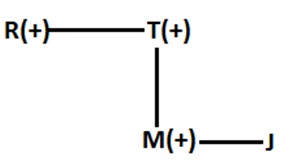QUESTION: 15

If ‘A × D’ means ‘A is the sister of D’, ‘A + D’ means ‘D is the daughter of A’ and ‘A ÷ D’ means ‘A is the mother of D’, then how will ‘N is the aunt of M’ be denoted?

Solution: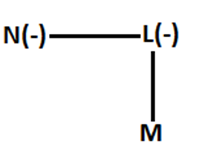QUESTION: 16

Directions: Study the following information carefully and answer the questions given below:

E, F, G, H, I, J and K are seven friends travelling in three different vehicles – Alto, Nano and Santro – with at least two in each vehicles. Each of them has a favourite cold drink, viz Frooti, Maaza, Slice, Sprite, Pepsi, Mirinda and 7Up but not necessarily in the same order.

I’s favourite drink is 7Up and F is travelling in Nano only with I. Those who travel in Alto do not like Frooti and Sprite. The one who likes Maaza travels only with K in Santro. The one whose favourite drink is Pepsi does not travel in the same vehicle as I or K. E does not travel in Nano but likes Mirinda. H and J are travelling in the same vehicle but J does not like Slice. The one whose favourite drink is Sprite does not travel in Nano.

Q.

Whose favourite drink is Maaza?

Solution: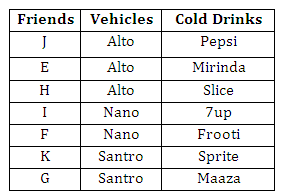QUESTION: 17

Directions: Study the following information carefully and answer the questions given below:

E, F, G, H, I, J and K are seven friends travelling in three different vehicles – Alto, Nano and Santro – with at least two in each vehicles. Each of them has a favourite cold drink, viz Frooti, Maaza, Slice, Sprite, Pepsi, Mirinda and 7Up but not necessarily in the same order.

I’s favourite drink is 7Up and F is travelling in Nano only with I. Those who travel in Alto do not like Frooti and Sprite. The one who likes Maaza travels only with K in Santro. The one whose favourite drink is Pepsi does not travel in the same vehicle as I or K. E does not travel in Nano but likes Mirinda. H and J are travelling in the same vehicle but J does not like Slice. The one whose favourite drink is Sprite does not travel in Nano.

Q.

Which of the following is the favourite drink of H?

Solution:QUESTION: 18

Directions: Study the following information carefully and answer the questions given below:

E, F, G, H, I, J and K are seven friends travelling in three different vehicles – Alto, Nano and Santro – with at least two in each vehicles. Each of them has a favourite cold drink, viz Frooti, Maaza, Slice, Sprite, Pepsi, Mirinda and 7Up but not necessarily in the same order.

I’s favourite drink is 7Up and F is travelling in Nano only with I. Those who travel in Alto do not like Frooti and Sprite. The one who likes Maaza travels only with K in Santro. The one whose favourite drink is Pepsi does not travel in the same vehicle as I or K. E does not travel in Nano but likes Mirinda. H and J are travelling in the same vehicle but J does not like Slice. The one whose favourite drink is Sprite does not travel in Nano.

Q.

In which of the following vehicles are three persons travelling?

Solution:QUESTION: 19

Directions: Study the following information carefully and answer the questions given below:

E, F, G, H, I, J and K are seven friends travelling in three different vehicles – Alto, Nano and Santro – with at least two in each vehicles. Each of them has a favourite cold drink, viz Frooti, Maaza, Slice, Sprite, Pepsi, Mirinda and 7Up but not necessarily in the same order.

I’s favourite drink is 7Up and F is travelling in Nano only with I. Those who travel in Alto do not like Frooti and Sprite. The one who likes Maaza travels only with K in Santro. The one whose favourite drink is Pepsi does not travel in the same vehicle as I or K. E does not travel in Nano but likes Mirinda. H and J are travelling in the same vehicle but J does not like Slice. The one whose favourite drink is Sprite does not travel in Nano.

Q.

Which of the following combinations is/true?

Solution:QUESTION: 20

Directions: Study the following information carefully and answer the questions given below:

E, F, G, H, I, J and K are seven friends travelling in three different vehicles – Alto, Nano and Santro – with at least two in each vehicles. Each of them has a favourite cold drink, viz Frooti, Maaza, Slice, Sprite, Pepsi, Mirinda and 7Up but not necessarily in the same order.

I’s favourite drink is 7Up and F is travelling in Nano only with I. Those who travel in Alto do not like Frooti and Sprite. The one who likes Maaza travels only with K in Santro. The one whose favourite drink is Pepsi does not travel in the same vehicle as I or K. E does not travel in Nano but likes Mirinda. H and J are travelling in the same vehicle but J does not like Slice. The one whose favourite drink is Sprite does not travel in Nano.

Q.

Which of the following statements is/are true?

Solution:QUESTION: 21

Directions: In the following questions, two Conclusions are given and five statements are given as (a), (b), (c), (d) and (e). From this you have to take the statements to be true even if they seem to be at variance with commonly known facts and then decide which of the given statement logically follows.

Q.

Conclusions:

All Donkeys being Tigers is a possibility

All Monkeys being Tigers is a possibility

Statements:

Solution: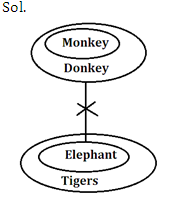QUESTION: 22

Directions: In the following questions, two Conclusions are given and five statements are given as (a), (b), (c), (d) and (e). From this you have to take the statements to be true even if they seem to be at variance with commonly known facts and then decide which of the given statement logically follows.

Q.

Conclusions:

Some covers are pillows.

All sheets being cover is a possibility.

Statements:

Solution: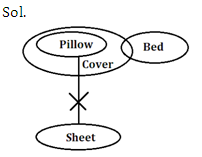QUESTION: 23

Directions: In the following questions, two Conclusions are given and five statements are given as (a), (b), (c), (d) and (e). From this you have to take the statements to be true even if they seem to be at variance with commonly known facts and then decide which of the given statement logically follows.

Q.

Conclusions:

All tanks are gingers.

Some gingers are not fishes

Statements:

Solution: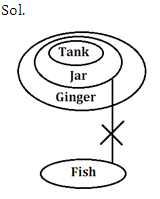QUESTION: 24

Directions: In the following questions, two Conclusions are given and five statements are given as (a), (b), (c), (d) and (e). From this you have to take the statements to be true even if they seem to be at variance with commonly known facts and then decide which of the given statement logically follows.

Q.

Conclusions:

Some elephants are being cats is a possibility

Some tigers are being elephants are a possibility

Statements:

Solution: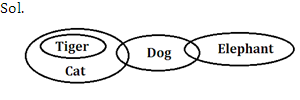QUESTION: 25

Directions: In the following questions, two Conclusions are given and five statements are given as (a), (b), (c), (d) and (e). From this you have to take the statements to be true even if they seem to be at variance with commonly known facts and then decide which of the given statement logically follows.

Q.

Conclusions:

Some mugs are plates

At least some trays are cups

Statements:

Solution: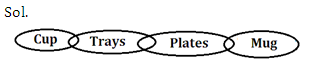QUESTION: 26

Directions : Study the information below and answer the following question:

In a certain code language,

‘Thin paper neatly folded’ is written as @D6, %R5, !N4, ?Y6

‘Four people from USA’ is written as @M4, %E6, #A3, @R4

‘Urban development programme launched’ is written as %E9, *T11, #N5,&D8

‘Dhaya likes forties hero’ is written as @E7, &S5, *A5, \$O4

Q.

The code for the word 'People' is-

Solution:
QUESTION: 27

Directions : Study the information below and answer the following question:

In a certain code language,

‘Thin paper neatly folded’ is written as @D6, %R5, !N4, ?Y6

‘Four people from USA’ is written as @M4, %E6, #A3, @R4

‘Urban development programme launched’ is written as %E9, *T11, #N5,&D8

‘Dhaya likes forties hero’ is written as @E7, &S5, *A5, \$O4

Q.

The code ‘*A5’ denotes which of the following word?

Solution:
QUESTION: 28

Directions : Study the information below and answer the following question:

In a certain code language,

‘Thin paper neatly folded’ is written as @D6, %R5, !N4, ?Y6

‘Four people from USA’ is written as @M4, %E6, #A3, @R4

‘Urban development programme launched’ is written as %E9, *T11, #N5,&D8

‘Dhaya likes forties hero’ is written as @E7, &S5, *A5, \$O4

Q.

The code word of ‘Four’ is

Solution:
QUESTION: 29

Directions : Study the information below and answer the following question:

In a certain code language,

‘Thin paper neatly folded’ is written as @D6, %R5, !N4, ?Y6

‘Four people from USA’ is written as @M4, %E6, #A3, @R4

‘Urban development programme launched’ is written as %E9, *T11, #N5,&D8

‘Dhaya likes forties hero’ is written as @E7, &S5, *A5, \$O4

Q.

‘#’ denotes which letter of the given words?

Solution:
QUESTION: 30

Directions : Study the information below and answer the following question:

In a certain code language,

‘Thin paper neatly folded’ is written as @D6, %R5, !N4, ?Y6

‘Four people from USA’ is written as @M4, %E6, #A3, @R4

‘Urban development programme launched’ is written as %E9, *T11, #N5,&D8

‘Dhaya likes forties hero’ is written as @E7, &S5, *A5, \$O4

Q.

According to the given code word, what will be the code for ‘Data Line reach points’?

Solution: